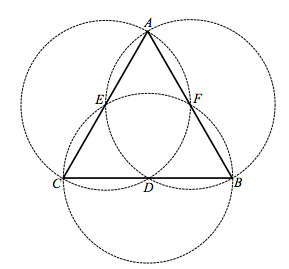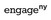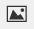PSA2QHK - Classwork---Geometry, M1, Lesson 3 (G.CO.1, G.CO.12)

In the following figure, circles have been constructed so that the endpoints of the diameter of each circle coincide with the endpoints of each segment of the equilateral triangle.

What is special about points D, E, and F? Explain how this can be confirmed with the use of a compass.Modified from EngageNY ©Great Minds Disclaimer

Part A)

In the following figure, circles have been constructed so that the endpoints of the diameter of each circle coincide with the endpoints of each segment of the equilateral triangle.

Draw, and. What kind of triangle mustbe?Modified from EngageNY ©Great Minds Disclaimer

Select one:
Part B)

Draw your drawing on paper, take a picture, and upload it using the image upload icon.

If you do not have the ability to upload an image of your work, type "Drawing is on paper."

In the following figure, circles have been constructed so that the endpoints of the diameter of each circle coincide with the endpoints of each segment of the equilateral triangle.

What is special about the four triangles within?Modified from EngageNY ©Great Minds Disclaimer

In the following figure, circles have been constructed so that the endpoints of the diameter of each circle coincide with the endpoints of each segment of the equilateral triangle.

How many times greater is the area ofthan the area of?Modified from EngageNY ©Great Minds Disclaimer

Type your answer below as a number (example: 5, 3.1, 4 1/2, or 3/2):